Robust Logistic and Probit Methods for Binary and Multinomial Regression | OMICS International
Journal of Biometrics & Biostatistics

Like us on:

All submissions of the EM system will be redirected to Online Manuscript Submission System. Authors are requested to submit articles directly to Online Manuscript Submission System of respective journal.

# Robust Logistic and Probit Methods for Binary and Multinomial Regression

Tabatabai MA1, Li H2, Eby WM3, Kengwoung-Keumo JJ2, Manne U4, Bae S5, Fouad M5 and Singh KP5*

1School of Graduate Studies and Research, Meharry Medical College, Nashville, TN 37208, USA

2Department of Mathematical Sciences, Cameron University, Lawton, OK 73505, USA

3Department of Mathematics, New Jersey City University, Jersey City, NJ 07305, USA

4Department of Pathology and Comprehensive Cancer Center, University of Alabama at Birmingham, Birmingham, AL 35294, USA

5Department of Medicine Division of Preventive Medicine and Comprehensive Cancer Center, University of Alabama at Birmingham, Birmingham, AL 35294, USA

*Corresponding Author:
Karan P Singh
Department of Medicine Division of Preventive Medicine
and Comprehensive Cancer Center
University of Alabama at Birmingham, Birmingham, AL 35294, USA
Tel: 205-934-6887
Fax: 205-934-4262
E-mail: [email protected]

Received date: July 01, 2014; Accepted date: July 27, 2014; Published date: July 30, 2014

Citation: Tabatabai MA, Li H, Eby WM, Kengwoung-Keumo JJ, Manne U, et al. (2014) Robust Logistic and Probit Methods for Binary and Multinomial Regression. J Biomet Biostat 5:202. doi:10.4172/2155-6180.1000202

Copyright: © 2014 Tabatabai MA, et al. This is an open-access article distributed under the terms of the Creative Commons Attribution License, which permits unrestricted use, distribution, and reproduction in any medium, provided the original author and source are are credited.

Visit for more related articles at Journal of Biometrics & Biostatistics

#### Abstract

In this paper we introduce new robust estimators for the logistic and probit regressions for binary, multinomial, nominal and ordinal data and apply these models to estimate the parameters when outliers or influential observations are present. Maximum likelihood estimates don’t behave well when outliers or influential observations are present. One remedy is to remove influential observations from the data and then apply the maximum likelihood technique on the deleted data. Another approach is to employ a robust technique that can handle outliers and influential observations without removing any observations from the data sets. The robustness of the method is tested using real and simulated data sets.

#### Introduction

Binary and multinomial regressions are commonly used by medical scientists and researchers for analysis of binary or polytomous outcomes. These methods are routinely used as diagnostic tools in all areas of medicine including oncology and cardiology. Zhou et al.  used logistic regression to relate the gene expression with class labels. They also used logistic regression for their microarray-based analysis of cancer classification and prediction. Sator et al.  applied a logistic regression model to identify enriched biological groups in gene expression microarray studies. Majid et al.  performed logistic regression analysis to predict endoscopic lesions in iron deficiency anemia when there are no gastrointestinal symptoms.

Morris et al.  applied multinomial regression technique to analyze the sub-phenotypes by allowing for heterogeneity of genetic effects. Richman et al.  investigated the association between European ancestry and renal disease when compared with African Americans, East Asians, and Hispanics. They concluded that European ancestry is protective against the development of renal disease in systematic lupus erythematosus. Their data had some outliers but they were excluded in their final analysis. Timmerman et al.  used the logistic regression to distinguish between benign and malignant adnexal mass before surgery. Merritt et al.  used the binary and multinomial logistic regressions to investigate the role of dairy food intake and risk of ovarian cancer. The validity of estimation and testing procedures used in the analysis of binary data are heavily dependent on whether or not the model assumptions are satisfied. The maximum likelihood method of estimating binary regression parameters using logistic, probit and many other methods is extremely sensitive to outliers and influential observations.

There is a large literature on the robustness issue of the binary regression. Most of the existing methods attempt to achieve robustness by down weighting observations which are far from the majority of the data, that is, outliers. The reader is referred to papers published by Pregibon , Carroll and Pederson , and Bianco and Yohai . Bianco and Martinez  modified the original score functions of the logistic regression to obtain bounded sensitivity, which is a concept introduced by Morgenthaler  using the L1-norm instead of the L2-norm in the likelihood, resulting in a weighted score function of the original score function. Cantoni and Ronchetti  focused on robustness of inference rather than the model. Pregibon  suggested resistant fitting methods which taper the standard likelihood to reduce the influence of extreme observations. Kordzakhia et al.  introduced a robust logistic regression by minimizing the mean-squared deviance for the worst case contamination. Bergesio and Yohai  introduced projection estimators for generalized linear model. These estimators have the same asymptotic normal distribution as the M-estimators. Hobza et al.  introduced a median estimator to estimate the parameters of the logistic regression.

Robust binary and multinomial regression estimators for analysis of biomedical data are proposed. This robust method has a bounded influence and high breakdown point and efficiency under normal distribution and is able to estimate the parameters of logistic and probit regression models. The proposed model is computationally simple and can easily be used by researchers.

#### Binary Regression Model

Consider the model yi=π(xi;β)+εi where ε12,…, εn are independent random variables with E(εi)=0 and Var(εi)=π(xi;β)(1−π(xi;β)), and y1,y2,…,yn are n independent Bernoulli random variables with Eyi=π(xi;β) and Var(yi)=π(xi;β)(1−π(xi;β)) such that the conditional success probability is given by P(yi=1| xi)=π(xi;β) and xi=(xi0,xi01,… ,xip)t; 1≤i≤n is a p+1 dimensional vector of predictor variables with β=(β01,…,βp)t as the parameters vector.

There are various estimation methods for the estimation of the parameter vector β. The most commonly used method is the logistic regression which is used to analyze the effects of explanatory variables on the binary response y. In the logistic regression the link function πL(xi;β) is assumed to have the following functional form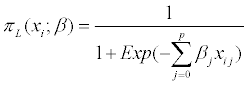The logistic transformation of πL(xi;β) is called the logit function and is given by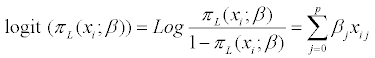The probit function is a link function of the form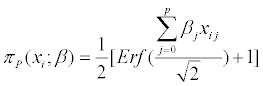Where Erf function is defined as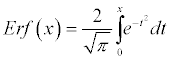which has the probit transformation function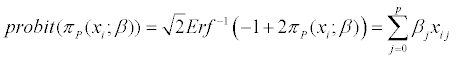The tabaistic model introduced by Tabatabai and Argyros  has the link function: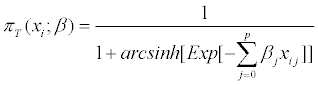Where arcsinh (.) represents the inverse hyperbolic sine function.

The tabaistic transformation function is called the tabit function and is defined as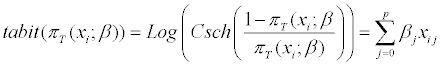Where Csch (.) denotes the hyperbolic cosecant function and the complementary log-log model link function has the form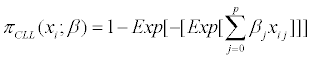with the complementary log-log transformation function (cllogit) defined as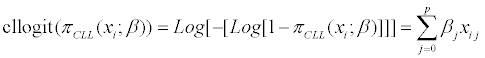Figure 1 shows the graph of πL, πCLL, πP and πT where of πL, πCCL, πP and πT take values between zero and one. The solid curve is the graph of πL function, the dotted curve is the graph of πP function, the dot-dashed curve is the graph of πCLL function, and the dashed curve is the graph of πT function. Figure 2 shows the graph of logit (πL). cllogit (πCLL), probit(πP), and tabit(πT). The solid curve, the dotted curve, the dotdashed curve and the dashed curve are the graph of logit (πL) function, the graph of probit(πP) function, the graph of cllogit (πCLL) function, and the graph of tabit(πT) function, respectively. The principle of maximum likelihood is ordinarily used to estimate the model parameters by maximizing the log-likelihood function of the form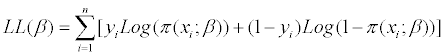Figure 2: Graphs of logit (πL). cllogit (πCLL), probit (πP), and tabit (πT) functions.

In other words, the estimate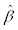of β is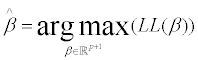Although the maximum likelihood estimator is asymptotically efficient, it is not recommended as a method of choice when outliers are present. The alternative techniques are robust statistical methods.

Tabatabai et al.  defined the one parameter family of differentiable functions ρω(x) of the form ρω(x)=1−Sech(ωx), where the positive real number ω is called the tuning constant.

The bounded function ρω: R→ R is a differentiable function satisfying the following properties: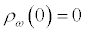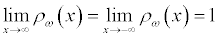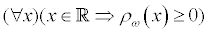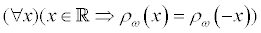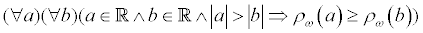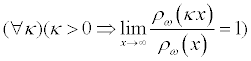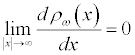Under the normality assumption for the error term εi, the asymptotic efficiency (Aeff) is defined as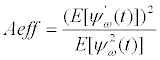(1)

Where ψω is the derivative of ρω and is equal to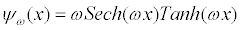Where Sech and Tanh represent the hyperbolic secant and hyperbolic tangent, respectively.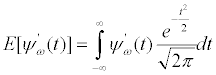with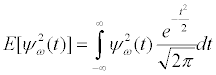The tuning constant ω can be calculated by solving the following equation (1) for ω. The numerical values for ω at the efficiency levels 0.80, 0.85, 0.90, and 0.95 are approximately 0.721, 0.628, 0.525 and 0.405, respectively. Although the choice for tuning constant ω is left for the investigator to decide, we do recommend an efficiency of approximately 90 percent which corresponds to ω=1/2. We now consider the hat matrix of the form

H = X(XtX)-1Xt,

where X is the design matrix defined as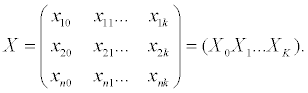If the model has intercept, then the column vector X0 has the form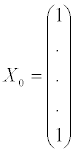For j=1,2,…,k, define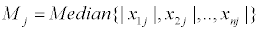and for i=1,2,…,n, define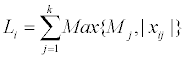and for ω>0 define the function Gω(u) as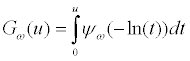(2)

The estimator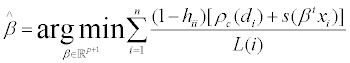(3)

Where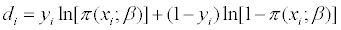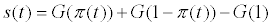and hii is the ith diagonal element in the hat matrix. For the logistic model, we have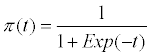So that the above integral (2) can be written as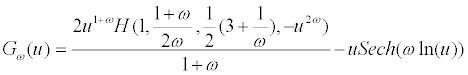Where H(k1,k2,k3,t) is the Gauss hypergeometric function 2F1 with parameters k1,k2 and k3. If ω=1, then we have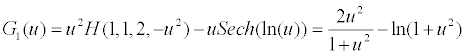and if ω=1/2, then we have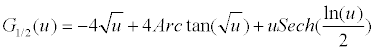Define the Hessian matrix Hb for binary data as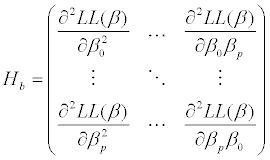Then an estimate of the variance-covariance matrix for vectoris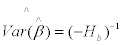with an estimated variance σ2 given by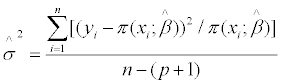To perform hypothesis testing, we let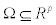be the parameter space and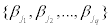be a subset of {β01,…,βp}. Define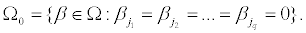To test the following hypothesis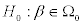against the alternative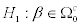one can use the Wald type test statistic which is defined as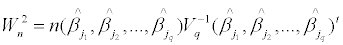The null distribution of the statistic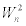is asymptotically a chisquare distribution with q degrees of freedom.

#### Robust Multinomial Logistic Regression Model

In this section we generalize the robust binary method to multinomial regression where the response y includes k categories. When k=2, this model reduces to the binary regression. Now, consider the response matrix Y given by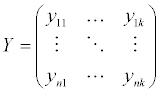where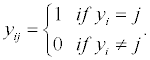This means that yij=1whenever the ith response is in category j.

For i =1,2,…,n,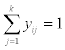and xi=(xi1,xi2,…,xip)t. The parameter vector

βj=(βj0j1,…,βjp)t and the vector β0 is a zero vector in a p+1 dimensional space with β=(β01,…,βk-1)t. The multinomial likelihood function of parameter vector β is defined by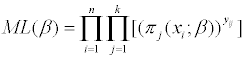and the multinomial log- likelihood function is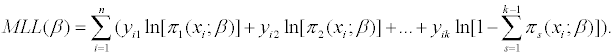For the generalized logit when the first category is the designated reference category and the intercepts are β0102,…,β0k-1, we get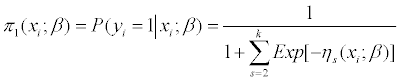and for j = 2,…,k,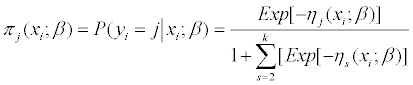and for j = 1,…,k−1 the logit function ηj(xj;β) is the log-odds of membership in category j versus the reference category 1 and is equal to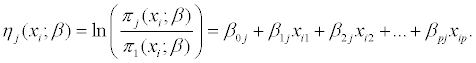The principle of maximum likelihood can be used to estimate model parameters. The maximum likelihood estimate of the parameters vector β is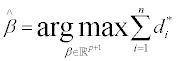(4)

where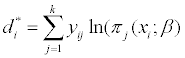The robust estimate of the model parameters is given by(5)

Define the Hessian matrix Hp as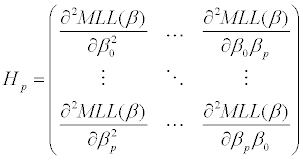Then, for the multinomial response, an estimate of the variancecovariance matrix for vectoris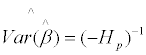with an estimated variance σ2 given by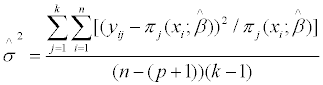For the cumulative logit model for ordinal k-category response, the cumulative probability for the ith response belongs to the response category less than or equal j is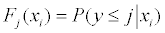and for j=1,…,k−1 the ordinal logit Oj(xj;β) is the log-odds of falling into or below category j against falling above it and is given by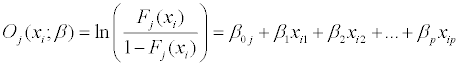where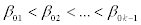. The ordered logit model is sometimes called the proportional odds model.

Let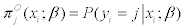. Then we have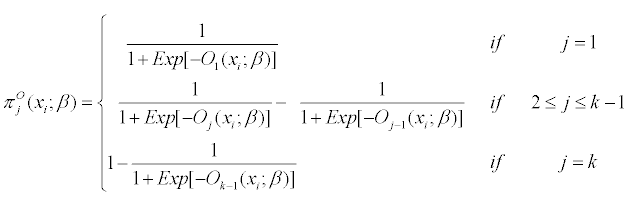and for the ordinal probit we have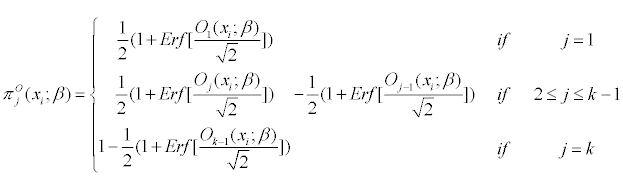The robust estimate of ordinal multinomial parameter vector is giveb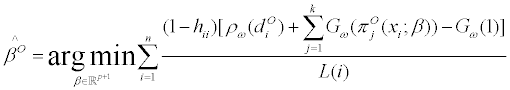(6)

where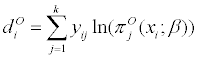#### Application

Vasoconstriction example

Vasoconstriction and vasodilation are two important physiological mechanisms used to control the circulation of blood throughout the body. These mechanisms directly affect both the blood pressure and the distribution of the blood in the body. Vasodilation refers to the expansion of blood vessels through relaxation of smooth muscles in the vessel walls. This allows increased flow of blood through these vessels and also decreases the blood pressure. Contraction of the same muscles tightens the blood vessels, which decreases blood flow and increases pressure. Thus, vasodilation and vasoconstriction work in opposition to adjust both blood flow and blood pressure. The usual controls for vasoconstriction and vasodilation are done by smooth muscles and autonomic nervous system, triggered by the medulla. These responses can also be affected by drugs promoting either constriction or dilation. Furthermore, there is a means of control by circulating hormones in the bloodstream, as well as control by intrinsic mechanisms to vasculature, called the myogenic response. The antagonistic operation of vasoconstriction and vasodilation is used by the body for numerous purposes. Primary among these is regulation of the supply of oxygen and nutrients to the cells of the body, to meet their needs. Furthermore, this regulation of blood flow is also needed for thermoregulation within the body. At times of increased metabolic needs or needs for oxygen in certain organs or systems in the body, the blood flow to these regions will also be modulated. Finally, vasoconstriction is also important in restricting blood flow to regions of the body in cases of traumatic injury.

The data set was analyzed originally by Finney . It consists of 39 observations where the binary response variable y=1 or y=0 represent the presence or absence of vasoconstriction of the skin respectively. This experimental data set considers the effect of inhalation of air in a single deep breath on the presence or absence of vasoconstriction in the digits. Presence or absence of vasoconstriction is considered as a categorical variable, and the study considers the effect of two variables, the volume of inhaled air and the rate of inhalation. (Data has hidden outliers so that the robust logistic regression will be useful in its analysis.) In the remainder of this work, we denote by ML and BY, the Maximum Likelihood and Bianco-Yohai methods, respectively. By examining Table 1 we conclude that the new robust estimator has produced the closest parameter estimates to the maximum likelihood estimates when the outliers were removed. In addition, the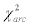is the lowest for the new robust method. Theis defined by Kordzakhia et al.  as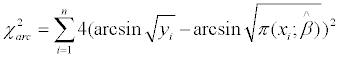Methods Coefficients
b0  b1 b2
Standard Error
b0 b1 b2
ML
ML (Influential observations
removed )
-2.887 5.191 4.578
(=48.3118)
-24.590 39.539 31.928
(=32.0159)
1.324 1.869  1.843

13.974  23.153 17.687
BY
c=1.25
-5.3214 8.44547.4801
(=43.1853)
9.7647 14.1903 12.3199

New Robust
c=0.5
-24.1191 38.4639 30.9608
(=32.2671)
15.3232 24.9410 19.2365

Table 1: Parameter estimates for Vaso Data Using ML, BY and New Robust Method.

Plasma example

The erythrocyte sedimentation rate (ESR) is very important. It is a common hematology test which is simple and inexpensive but can be used to detect infection or acute phase response, which can alert physicians to a wide variety of conditions. The test is very versatile and can assist physicians in detecting conditions from rheumatoid arthritis to systemic lupus erythematosus to multiple myeloma; however it is non-specific and is usually combined with other tests. In practice, ESR is used widely to test for a range of conditions, including inflammation, trauma, and malignant disease. Studies have also suggested the utility of the ESR among the elderly as a general indicator of level of sickness or disease. Recently ESR has also attracted attention for a potential role as a predictor for the development of cardio-vascular disease and heart failure.

The ESR simply measures the rate at which red blood cells precipitate during a period of one hour. Anticoagulated blood is placed in an upright tube, and the rate at which the erythrocytes settle is measured in mm per hour. Although the test is a direct measurement of rate of sedimentation, the balance between factors stimulating sedimentation and factors resisting sedimentation allows for a number of clinically relevant factors to influence this rate. Fibrinogen is the most important factor promoting sedimentation, and the high level of fibrinogen in the blood during the inflammatory process makes this test sensitive to inflammation. High levels of fibrinogen in the blood decrease the repulsive forces experienced between the negatively charged erythrocytes and favor the formation of rouleaux. These stacks of erythrocytes that stick together will settle faster and lead to an increased ESR. Other acute phase reactants, or other large molecules, especially when positively charged, can have a similar effect, although fibrinogen has been observed to have the largest effect.

A recent focus on the inflammatory nature of artherosclerosis has been accompanied by a recent study of increased levels of ESR and elevated risk of coronary heart disease. Erikssen et al.  observed that elevated ESR is a strong predictor of mortality from heart failure, suggesting it may serve as a marker for aggressive forms of coronary heart disease. Andresdottir et al.  observed an increased risk of coronary heart disease among the top quintile or ESR rates, with a hazard ratio of 1.57 for men and 1.9 for women. The 2005 paper of Inglesson et al.  also observes a significant association between elevated ESR and heart failure, suggesting both that inflammation is involved in the processes leading to heart failure and that the ESR may be used in evaluating this process. In addition to the well-established uses of ESR, Saadeh  mentions some potential new applications of this test such as bacterial otitis media, acute hematogenous osteomyelitis, AIDS, pelvic inflammatory disease, prostate cancer, and early prediction of stroke severity.

Although the ESR usually detects acute phase response from fibrinogen in blood in conditions such as those mentioned above, in certain cases there are factors which decrease the rate of sedimentation. One important factor that can slow the rate of sedimentation is irregularity in the erythrocytes, either in shape or unusually small size. As a consequence, ESR can detect certain blood diseases (including sickle cell anemia and spherocytosis) which lead to a lower than normal rate of sedimentation, as observed in Bridgen . Other conditions that may also lower ESR include the extreme levels of white blood cells as observed in chronic lymphocytic leukemia. Furthermore the surplus of erythrocytes found in patients with polycythemia makes rouleau formation difficult and decreases the ESR.

In clinical applications the erythrocyte sedimentation rate may in many cases be treated as a categorical variable, with a normal ESR for values less than some given α and an elevated ESR for values greater than α. When representing such a set of data where ESR depends on one or more variables the logistic regression may be used. For instance in the data set from Collett , the ESR is considered as a function of two variables, the level of fibrinogen and the level of γ-globulin. The data for 32 individuals represents the levels of fibrinogen and γ-globulin in the blood and whether the ESR level is healthy (< 20 mm/hr) or unhealthy (≥ 20 mm/hr), and the logistic regression is used to describe how both fibrinogen and γ-globulin affect the ESR variable. Since this data set contains (hidden/influential) outliers, both the probit method of regression and the logit method do not give accurate results. However we observed that our new methods for robust logistic regression do represent the data accurately. The logit, when all 32 observations are included in the study, is given by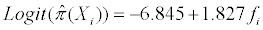When one removes the influential observations 15, and 23, the logit model becomes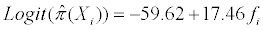The level of γ-globulin was not a statistically significant variable to be included in the model. Thus only the level of fibrinogen f is used in the variable selection.

Again, examining Table 2 reveals that the new robust estimator has produced the closest parameter estimates to the maximum likelihood estimates when the outliers were removed as well as the lowest value for theMethods Coefficients
b0 b1
Standard Error
b0 b1
ML

ML (Influential observations removed )
-6.8451 1.8271
(=38.9405)
-59.62 17.46
(=29.1415)
2.7703  0.9009

45.5113.50

BY
c=1.25
-8.3774 2.2870
(=37.0421)
5.4383  1.6632

New method
c=0.5
-60.5094 17.7654
(=29.2719)
49.7325  14.7409

Table 2: Parameter Estimates for Plasma Data Using ML, BY and New Robust Method.

Mental health example

The following example involves the ordinal multinomial regression. The data comes from a mental health study for a random sample of adult residents of Alachua County, Florida. This data was appeared in Agresti . The mental impairment is divided into four categories (well, mild symptom formation, moderate symptom formation, and impaired). The explanatory variables are life events index X1 and socioeconomic status X2, where X2 is binary and takes high and low levels. There is no outlier in this data. We just want to show how the method works even when outliers are not present. The logit is given by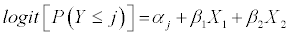Table 3 gives the parameter values using maximum likelihood method as well as the new robust ordinal multinomial method. The R program for this example is provided in the Appendix.

Parameter Estimated Standard Error Robust estimate Standard Error
Intercept 1 -0.2819 0.6423 -.2374 0.7265
Intercept 2 1.2128 0.6607 1.1923 0.7507
Intercept 3 2.2094 0.7210 2.2981 0.8364
Life -0.3189 0.1210 -.3181 0.1618
Socioeconomic status 1.1112 0.6109 1.1423 0.7789

Table 3: Parameter estimates for Mental Health data using robust ordinal method.

#### Simulation

To evaluate the performance of the new robust method for logistic regression we conduct a Monte Carlo simulation. In the first round of our simulation, we use one explanatory variable and in the next round, we increase the number of explanatory variables to two. We first generate an independent random sample of size 100 from the standard normal distribution with mean 0 and standard deviation equal to 0.5. We call the variable x. Then we generate a sample of error terms εi of size 100 from the logistic distribution with mean zero and standard deviation equal to 1. The dependent variable y is generated using the formula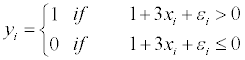The model parameters are 1 (intercept) and 3 (coefficient for x). We then select a random sample (5%) from the generated sample x and contaminate the selected sample by multiplying each x value by a factor of 10. Then we repeat the above procedures 1000 times. Finally, we estimate both the bias and mean squared errors using the following equations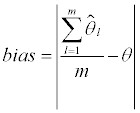where m is the number of iterations in the simulation. The mean squared error is estimated by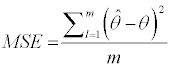For the two explanatory variables, we generate two independent normal random samples of size 100 from a normal distribution with mean 0 and standard deviation 0.5 and call them x1 and x2 respectively. Then we select 5% of this random sample (3% from x1 and 2% from x2) and multiply the selected samples by 10. Then we generate a sample of error terms εi of size 100 from the logistic distribution with mean zero and standard deviation equal to 1. The dependent variable y is generated using the formula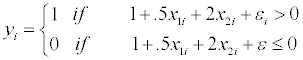We calculate the parameter estimates and continue the iteration 1000 times. In addition, we calculate the bias and mean squared errors. Tables 4 and 5 show the results of simulations using ML, BY and the new robust method with one and two explanatory variables, respectively. For binary logistic regression the simulation results indicate that our new robust method is as good as the BY method. The BY method only covers binary logistic regression whereas our method not only covers binary but also covers multinomial regression for both nominal and ordinal responses.

Method Bias MSE Bias  (5% x) MSE (5%)
ML
b0 0.0430 0.0876 0.08065 0.07938
b1 0.1784 0..6310 1.00777 2.16368
BY
b0 0.0463 0.0923 0.0027 0.0842
b1 0.1857 0.6662 0.2336 0.8885
New Robust
b0 0.0342 0.0897 0.0125 0.0779
b1 0.1125 0.5321 0.2642 0.9771

Table 4: Simulation Results for Logistic Regression (b0=1, b1=3, N=100, m=1000).

Method Bias MSE Bias (5%) MSE (5%)
ML
b0 0.04283 0.08152 0.00312 0.07526
b1 0.01071 0.25287 0.19770 0.23694
b2 0.10448 0.38209 0.36612 0.75513
BY
b0 0.0485 0.0856 0.0232 0.0788
b1 0.0128 0.2650 0.1711 0.2456
b2 0.1143 0.4028 0.0909 0.5125
New Robust
b0 0.0410 0.0786 0.0270 0.0755
b1 0.0255 0.2799 0.1045 0.2676
b2 0.1034 0.4037 0.0553 0.5032

Table 5: Simulation Results for Logistic Regression (b0=1, b1=0.5, b2=2, N=100, m=1000).

#### Discussion and Conclusions

In this work we have proposed a new robust method to analyze binary and multinomial regression models. We believe that these new robust methods for binary and multinomial regressions have potential to play a key role in modeling categorical data in medical, biological and engineering sciences. We have shown the lack of robustness of the maximum likelihood technique when outliers are present. In both real examples and simulated ones and when the outliers are present, the new robust method performed well. In conclusion the motivation was to introduce a new robust loss function of residuals which can attain high breakdown value. The method has high efficiency and high breakdown points with bounded influence function.

#### Acknowledgement

Research reported in this paper was partially supported by the Center grant of the National Cancer Institute of the National Institutes of Health to the University of Alabama at Birmingham Comprehensive Cancer Center (P30 CA013148), the Cervical SPORE grant (P50CA098252), the Morehouse/Tuskegee University/ UAB Comprehensive Cancer Center Partnership grant (2U54-CA118948), and the Mid-South Transdisciplinary Collaborative Center for Health Disparities Research (U54MD008176). The content is solely the responsibility of the authors and does not necessarily represent the official views of the National Institutes of Health.

#### References

Select your language of interest to view the total content in your interested language

### Article Usage

• Total views: 13485
• [From(publication date):
August-2014 - Nov 21, 2019]
• Breakdown by view type
• HTML page views : 9601Can't read the image? click here to refresh